Anzeige

# SECTION 1 - CHAPTER 4 - INTRODUCTION TO CHARTS - PART 2

22. Mar 2023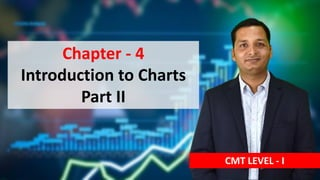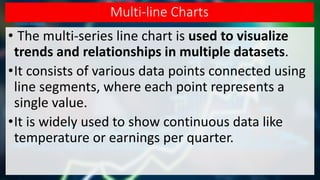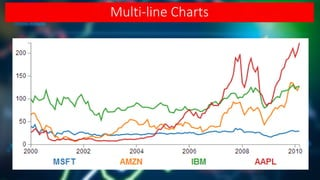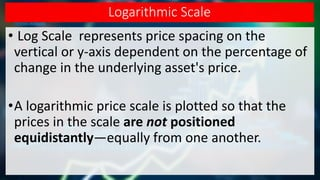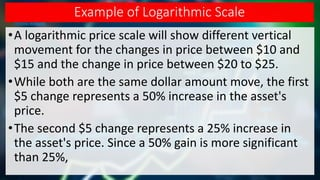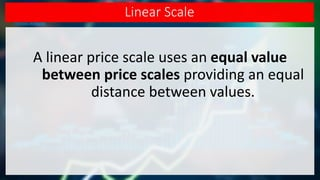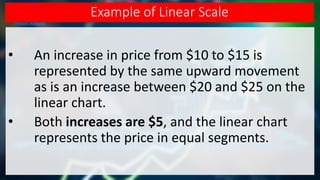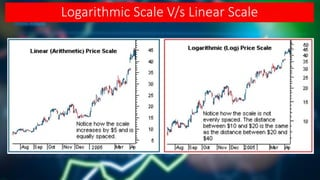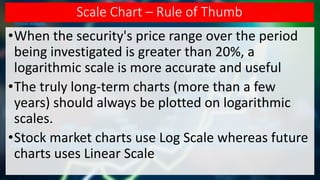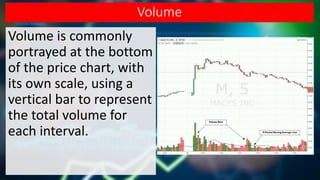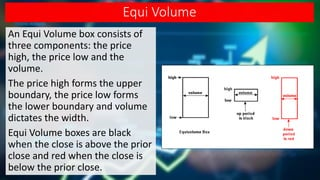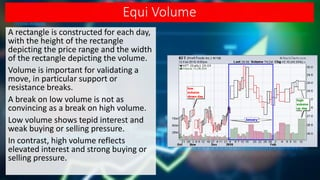1 von 13
Anzeige

### SECTION 1 - CHAPTER 4 - INTRODUCTION TO CHARTS - PART 2

1. Chapter - 4 Introduction to Charts Part II CMT LEVEL - I
2. Multi-line Charts • The multi-series line chart is used to visualize trends and relationships in multiple datasets. •It consists of various data points connected using line segments, where each point represents a single value. •It is widely used to show continuous data like temperature or earnings per quarter.
3. Multi-line Charts
4. Vertical Scale CMT LEVEL - I
5. Logarithmic Scale • Log Scale represents price spacing on the vertical or y-axis dependent on the percentage of change in the underlying asset's price. •A logarithmic price scale is plotted so that the prices in the scale are not positioned equidistantly—equally from one another.
6. Example of Logarithmic Scale •A logarithmic price scale will show different vertical movement for the changes in price between \$10 and \$15 and the change in price between \$20 to \$25. •While both are the same dollar amount move, the first \$5 change represents a 50% increase in the asset's price. •The second \$5 change represents a 25% increase in the asset's price. Since a 50% gain is more significant than 25%,
7. Linear Scale A linear price scale uses an equal value between price scales providing an equal distance between values.
8. Example of Linear Scale • An increase in price from \$10 to \$15 is represented by the same upward movement as is an increase between \$20 and \$25 on the linear chart. • Both increases are \$5, and the linear chart represents the price in equal segments.
9. Logarithmic Scale V/s Linear Scale
10. Scale Chart – Rule of Thumb •When the security's price range over the period being investigated is greater than 20%, a logarithmic scale is more accurate and useful •The truly long-term charts (more than a few years) should always be plotted on logarithmic scales. •Stock market charts use Log Scale whereas future charts uses Linear Scale
11. Volume Volume is commonly portrayed at the bottom of the price chart, with its own scale, using a vertical bar to represent the total volume for each interval.
12. Equi Volume An Equi Volume box consists of three components: the price high, the price low and the volume. The price high forms the upper boundary, the price low forms the lower boundary and volume dictates the width. Equi Volume boxes are black when the close is above the prior close and red when the close is below the prior close.
13. Equi Volume A rectangle is constructed for each day, with the height of the rectangle depicting the price range and the width of the rectangle depicting the volume. Volume is important for validating a move, in particular support or resistance breaks. A break on low volume is not as convincing as a break on high volume. Low volume shows tepid interest and weak buying or selling pressure. In contrast, high volume reflects elevated interest and strong buying or selling pressure.
Anzeige